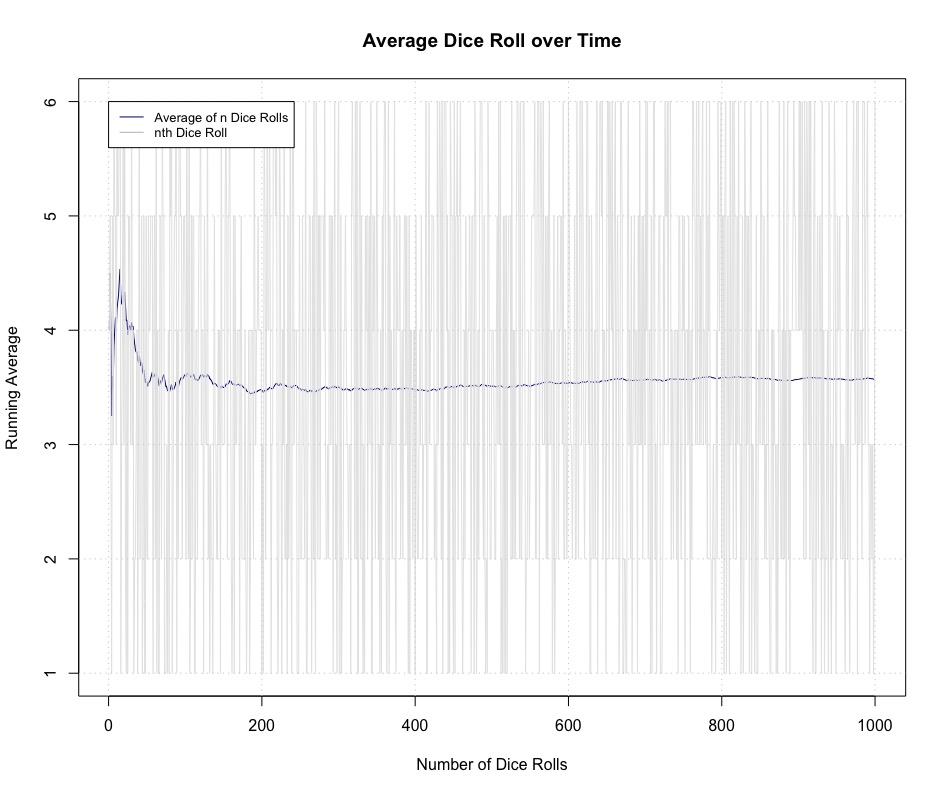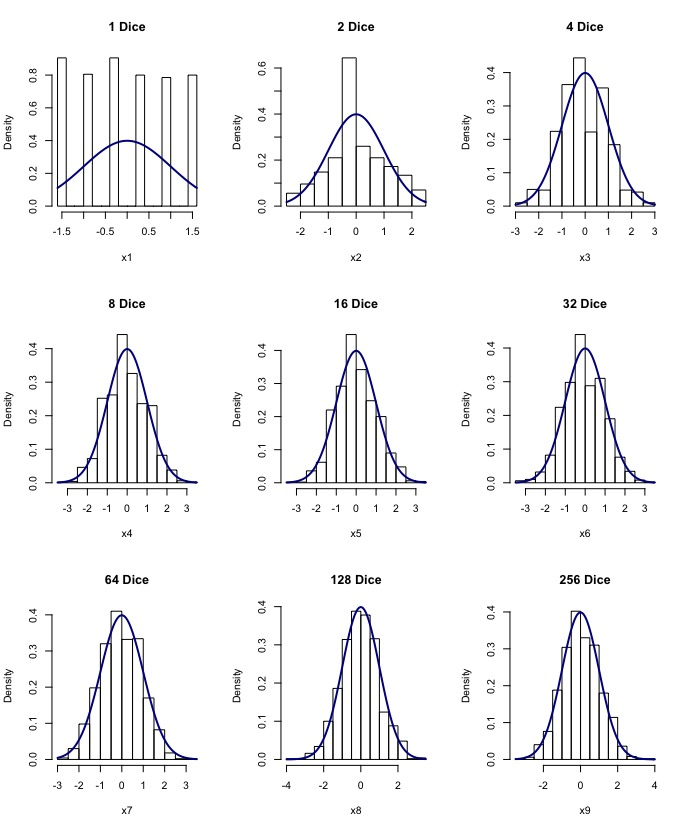By Hugh Entwistle, Macquarie University

In a world increasingly driven by data, the use of statistics to understand and analyse data is an essential tool. Behind most aspects of data analysis, the Central Limit Theorem will most likely have been used to simplify the underlying mathematics or justify major assumptions in the tools used in the analysis – such as in Regression models.

To get a first intuition for the Central Limit theorem, note that generally the average of a large sample of values, dice rolls for example, will tend to converge towards a certain number. This phenomenon is displayed in the following graph, and is mathematically referred to as the ‘Law of Large Numbers’.The Central Limit Theorem describes, however, how in most contexts, the distribution of large enough amounts of data will eventually follow the normal distribution – or more familiarly for the reader, a ‘bell curve’. This is a step further than the Law of Large Numbers – giving an insight into the actual behaviour of the sample as it gets larger, not just the eventual average. As the normal distribution is well understood by mathematicians, it is very useful for approximating distributions that would be harder to compute properties for. We can see this visually through simulation and standardising each sum, displaying the results in a histogram – the standard normal distribution curve is placed on top of each simulation to show the inevitable convergence to the normal distribution as the number of dice gets larger.However, the Central Limit Theorem is often misunderstood as it is applied in situations where the conditions may not be successfully met. For example, it cannot be applied to the Cauchy distribution – where it can be shown that the sum of Cauchy variables will always follow the same distribution and will never become normally distributed.

Thus my project involved looking at different versions of the Central Limit Theorem where different requirements such as independence and what distributions the variables may come from would vary, as well as looking at the conditions and demonstrating distributions where some of the conditions would not be met.

A question that naturally arises from this discussion is “How many observations will be required for this effect to manifest itself enough for us to start using the normal distribution to approximate the distribution that we are working with?”. Naturally, it can be appreciated that for some scientific experiments that this question could perhaps have ethical, environmental, economic or practical consequences depending on the nature of the data needed. Many textbooks and websites provide the answer as “Thirty”. This is very unsatisfying, so my project was motivated by answering this question on a more quantitative level. This question can be answered by the Berry Esseen Theorem which gives an upper bound on the maximum difference between the two distributions. This upper bound is in terms of the third absolute moment and the variance of the distribution – showing that the answer to the aforementioned question is: “Depends on the distribution”. We thus must be respectful of the distribution we are using before determining whether or not it is appropriate to use the Central Limit Theorem even if we do have thirty observations!

This upper bound, however, is still not precisely known as it contains a universal constant that is being continuously improved upon by mathematicians, with upper and lower bounds for this constant being found even in the past seven years – making it a relevant and exciting area of research, one of which I have barely skimmed the surface but I now have a better appreciation of a famous, often neglected, theorem in statistics after exploring some of the deeper nuances and properties of the underlying mathematics.

Hugh Entwistle was a recipient of a 2018/19 AMSI Vacation Research Scholarship.